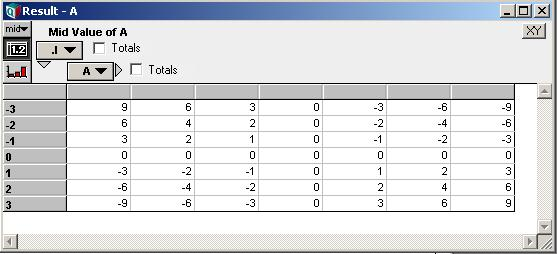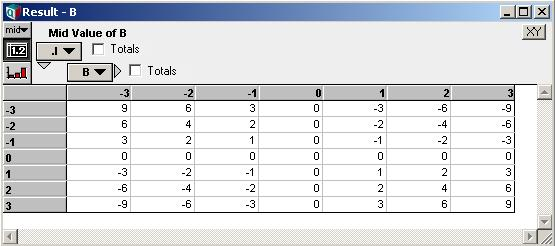# Implicit Dimensions

Each dimension of an Analytica array is defined by an index. Each dimension of an array is identified by a different index, and when expressions operate over an array, the index can be specified to indicate which dimension is being operated over. We say these indexes are explicit, since these indexes each have a name, giving us a way to identify the dimension of interest in an expression.

An array may also contain one implicit dimension. An implicit dimension has no explicit index, and so cannot be identified by name. Analytica does not allow more than one implicit index in an array, since it would create an ambiguity.

Implicit dimensions are sometimes also referred to as null-indexes, null-dimensions, or list-dimensions.

Although you cannot identify an implicit dimension by name, some Analytica functions that normally work over an identified index, can be applied across the implicit dimension using variations that omit the index parameter. For example, consider the expression

Max([x, y, z])

The intermediate expression, [x, y, z], evaluates to an array. The brackets introduce an implicit dimension, and if x, y, or z are themselves arrays, the result of [x, y, z] may have multiple dimensions. Because the second index parameter to Max is not specified, the maximum is computed across the implicit dimension.

## Multiple Implicit Dimensions Not Allowed

As we mentioned, an array can have at most one implicit dimension. If you try to combine two implicit dimensions, it will cause an error. For example, evaluating

(1..10) * (1..10)

generates this error message:

Trying to combine two arrays with implicit indexes. An array can contain only one implicit index. One cure might be to define at least one of these as an explicit index.

As the message implies, we can get around this limitation by defining two Local Indexes:

Index I := 1..10;
Index J := 1..10;
I * J

Note that Local Indexes are explicit indexes -- they have a name, and can be referred to outside the code in which they were defined if they persist in the result value.

## Promotion to Self-Index

If an implicit dimension still exists in the result after the full definition of a variable has been evaluated, the implicit dimension gets promoted to be a self index of the result variable. So once the value is stored, the result will no longer have an implicit dimension, since a self index is explicit. From other variables, the dimension can then be referred to by name.

Suppose Variable Scenario is defined as:

"Case " & (1..10)

When this expression is evaluated, a list (considered equivalent to a 1-D array with an implicit index) having elements:

["Case 1","Case 2",....,"Case 10"]

is computed. When this is stored as the value of Scenario, the implicit dimension becomes the index value for Scenario.

When an implicit index gets promoted to a Self index, the index element values may not be available at the time of promotion. This happens with the following example:

Index I := -3..3;
I * (-3..3)

The result of this expression is a 2-D array, with the dimension introduced by (-3..3) being implicit. When this 2-D value is stored, the implicit dimension becomes the self-index for the variable, but the index values for that dimension are all blank, with the result appearing asNotice the blank column labels for the self-index A. Because an implicit dimension is not associated with any Analytica object, the Analytica engine does not keep any index element values around for those values while evaluating is taking place, so that at the time of promotion, these aren't available. Thus, you cannot meaningful use Subscript on this self-index A -- access to specific rows would require the use of Slice. The dimension does, nevertheless, fully array abstract like any other explicit index. If you need the index element values, then use an explicit index.

There are two cases in which Analytica can recover element values when promoting an implicit index to a self index. First, if the result is one-dimensional, with the implicit index being the only dimension, then those values become the self-index element values. For example:

(2..5)^2

will evaluate to [4, 9,16, 25], and those squares will become the index values. Because it is 1-D, Analytica assumes the actual array values are the index values. The second case is when an explicit list, with the bracket notation, appears in a definition. For example:

Index I := -3..3;
I * [-3, -2, -1, 0, 1, 2, 3]

When brackets are used, Analytica uses the original values or expressions appearing inside the brackets as the index values. In this example, the result looks likeHere notice that the original values appear in the column headers.

When Analytica takes index values from explicit list notation (with brackets), it uses the original values or expressions appearing in the brackets as the index element values. Thus, notice the difference between the following two definitions:

(2..5)^2
[2, 3, 4, 5]^2

In the second case, the result array values are different from the self-index values, while in the first case they are the same.

There are several "gotchas" when using bracketed lists. If you include identifiers as elements in the list, the self-index value will contain Handles to objects. These can be referenced in subscript operations, e.g., as A[A = Handle(X)]. If your bracketed list contains expressions, the element values will be parsed expressions, which is a data structure not readily processed from within Analytica expressions. You may find yourself having to use Slice rather than Subscript when expressions (non-literals) are present. However, these expressions may simplify your life when viewing graphs of results, etc. Again, whenever you need more control over the precise labels that appear, use a local index rather than an implicit index.

## Implicit Dimensions with Local Variables

Local variables are declared using the Var..Do construct, as in:

Var X := 1..10 Do X^2

A local variable declared in this fashion is not exactly equivalent to a local index declared as

Index X := 1..10 Do X^2

The Var..Do construct treats X as an alias for the value 1..10, while the Index..Do construct actually creates a new Analytica index object. Thus, in the first case with Var..Do, X is an implicit dimension, while in the second case X is an explicit dimension.

However, when an implicit dimension is assigned to a local variable, within the lexical scope of that variable there is a way to refer to the dimension -- i.e., using the local variable's name. Hence, while the local variable remains in lexical scope, there is no longer an ambiguity if two implicit dimensions appear in the same array. Therefore, the following IS allowed:

Var X := 1..10;
Var Y := 1..10;
Sum(10*X + Y, Y)

In this expression, when 10*X + Y is evaluated, two implicit dimensions appear in the result; however, because they can still be referred to by name, an error is not issued. Analytica can figure out which implicit dimension the second parameter of Sum refers to.

On the other hand, in the expression

Var X := 1..10;
Var Y := 1..10;
10*X + Y

an error about multiple implicit dimensions will result. There is no problem when 10*X + Y is evaluated, since there isn't yet an ambiguity, but then the value is returned outside of the lexical scope of X and Y, at which point two implicit dimensions that cannot be referred to by name exist, and at that point, an error results.

## Operating over Implicit Dimensions

Analytica functions that operate over an array have one or more index parameters that specify which dimension is being operated over. Most of these functions allow variations in which the index parameter can be omitted. These variations are there to provide a way to operate over the implicit dimension.

For example:

Index X := 1..10;
Sum((1..10) * X^2)

The parameter to Sum here contains two dimensions, but since the second parameter to Sum, the index parameter, is not specified, the summation occurs over the implicit dimension. The general rule is that if there is an implicit dimension and index parameter is omitted, then the operation will occur over the implicit dimension.

Because a local variable defined using Var..Do is considered implicit (as opposed to a local variable defined using Index..Do, its dimension will take precedence if it appears with other dimensions. Thus in

Var X := 1..10;
Sum(X*10 + Time)

the summation will occur over the implicit dimension introduced by X.

We highly recommend explicitly specifying the index parameter in all cases where it is possible. Even though omission of index parameters is allowed by many Analytica functions, it is bad style to omit them unless you do intend to operate over the implicit dimension.

## Lists within references

As discussed above, lists or implicit dimensions get promoted to self-indexes if the implicit dimensions reach the top level. This, however, does not occur if the value is within a reference. So, for example, the implicit dimension in

\(1..10)

does not get promoted to a self-index. It remains a list when dereferenced, even from another variable's definition.

Using references, you can have multiple implicit dimensions, or multiple lists, stored within a single value. Because each is within its own reference, they do not directly interact. Lists stored in this fashion can be different lengths, etc.

One limitation here is that you cannot specify the null dimension to the reference operator -- telling it to "swallow" the implicit dimension. With explicit dimensions you can use write:

\[I, J]A

to tell the reference operator to "swallow" the I and J dimensions, but to leave any other dimensions outside the reference. Thus, to swallow an implicit dimension, you cannot specify any explicit indexes to the reference operator.

## User Defined Function Parameters

To create a user-defined function that operates over the implicit dimension, use the List qualifier. For example:

Fu1(L : List)

inside the function definition, L will contain only the implicit dimension. To include the implicit dimension along with other explicit dimensions, use e.g.:

Fu1(L: List[I, J]; I, J: Index)

Here L will be 3-D, with one dimension being implicit.